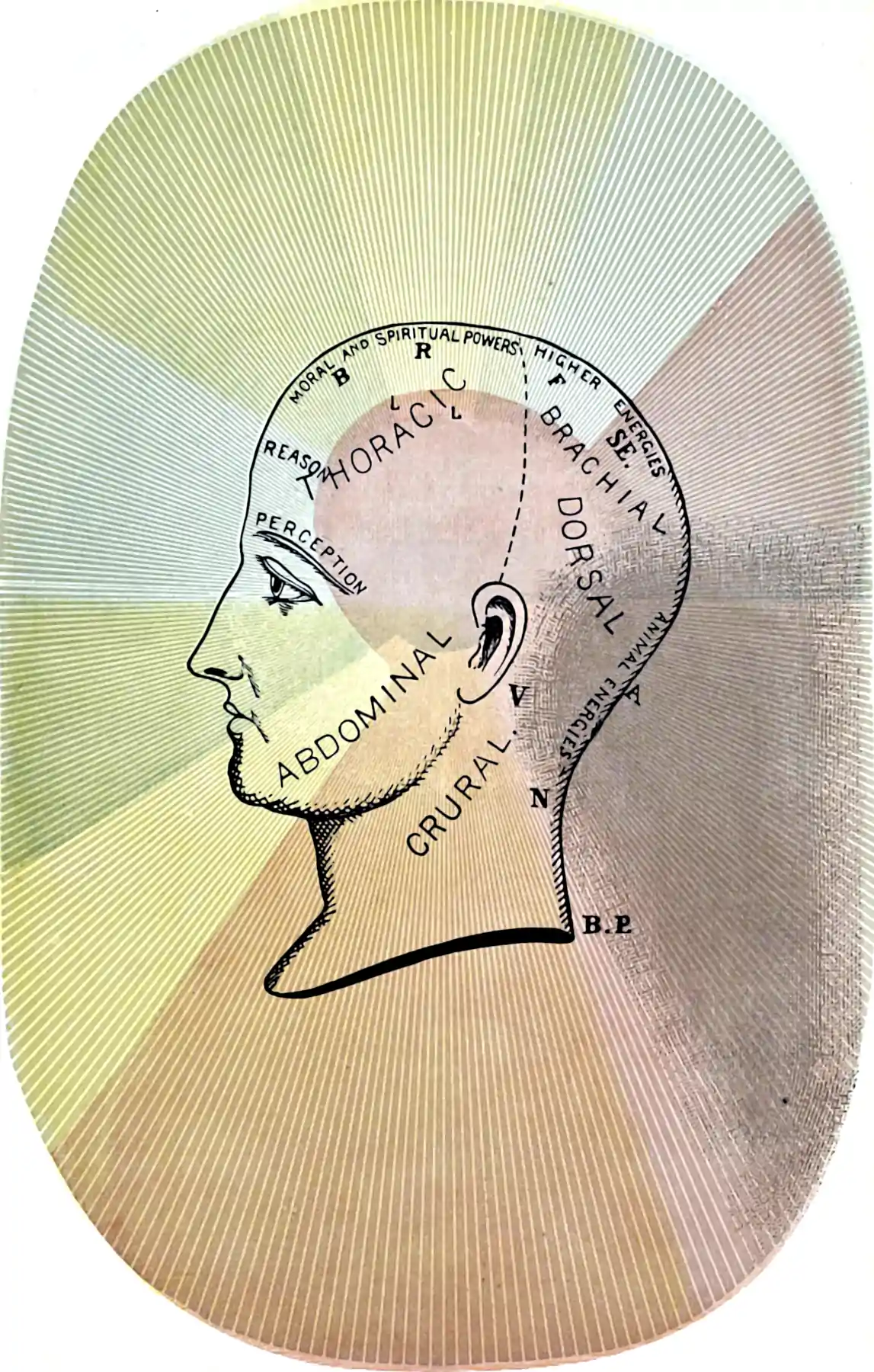# Algorithmic statistics

## Probably also algorithmic information theoryThe intersection between probability, ignorance, and algorithms, butting up against computational complexity, coding theory, dynamical systems, ergodic theory, minimum description length and probability. Random number generation relates here, too.

When is the relation between things sufficiently jointly unstructured that we may treat them as random? Stochastic approximations to deterministic algorithms. Kolmogorov complexity. Compressibility, Shannon information. Sideswipe at deterministic chaos. Chaotic systems treated as if stochastic. (Are “real” systems not precisely that?) Statistical mechanics and ergodicity.

I saw a provocative talk by Daniela Andrés on, nominally, Parkinson’s disease. The grabbing part was talking about the care and feeding of neural “codewords”, and the information theory of the brain, which she did in the foreign (to me) language of “algorithmic statistics”, and “Kolmogorov structure functions”. I have no idea what she meant. This is a placeholder to remind me to come back and see if it is as useful as it sounded like it might be.

To consider: relationship between underlying event space and measures we construct on such spaces. How much topology is lost by laundering our events through pullback of a (e.g. probability) measure?

Chazelle:

The discrepancy method has produced the most fruitful line of attack on a pivotal computer science question: What is the computational power of random bits? It has also played a major role in recent developments in complexity theory. This book tells the story of the discrepancy method in a few succinct independent vignettes. The chapters explore such topics as communication complexity, pseudo-randomness, rapidly mixing Markov chains, points on a sphere, derandomization, convex hulls and Voronoi diagrams, linear programming, geometric sampling and VC-dimension theory, minimum spanning trees, circuit complexity, and multidimensional searching. The mathematical treatment is thorough and self-contained, with minimal prerequisites. More information can be found on the book’s home page.

Cosma Shalizi’s upcoming textbook has the world’s pithiest summary:

In fact, what we really have to assume is that the relationships between the causes omitted from the DAG and those included is so intricate and convoluted that it might as well be noise, along the lines of algorithmic information theory , whose key result might be summed up as “Any determinism distinguishable from randomness is insufficiently complex”.

Cosma’s Algorithmic Information Theory notebook is also pretty good an extremely pithy.

• Just had Mezard and Montanari (2009) recommended by David Donoho. Online.

## Empirical estimation of computation

As far as I can tell, this is the main research thrust of the Max Planck institute at Leipzig to solve a kind of inverse problem, inspecting inferred probabilities for evidence of computation. Is that… sane? Why would one wish to do that?## Information-based complexity theory

Is a specialty within this field?

Information-based complexity (IBC) is the branch of computational complexity that studies problems for which the information is partial, contaminated, and priced.

To motivate these assumptions about information consider the problem of the numerical computation of an integral. Here, the integrands consist of functions defined over the d-dimensional unit cube. Since a digital computer can store only a finite set of numbers, these functions must be replaced by such finite sets (by, for example, evaluating the functions at a finite number of points). Therefore, we have only partial information about the functions. Furthermore, the function values may be contaminated by round-off error. Finally, evaluating the functions can be expensive, and so computing these values has a price.

• The RJLipton post on IBC linking it to complexity theory:

Now as ordinary complexity theorists, our first instinct would be to define properties intrinsic to the function $$\{f\}$$ and try to prove they cause high complexity for any algorithm. Making a continuous analogy to concepts in discrete Boolean complexity, drawing on papers like this by Noam Nisan and Mario Szegedy, we would try to tailor an effective measure of “sensitivity.” We would talk about functions $$\{f\}$$ that resemble the $$\{n\}$$-ary parity function in respect of sensitivity but don’t have a simple known integral. Notions of $$\{f\}$$ being “isotropic” could cut both ways—they could make the sensitivity pervasive but could enable a good global estimate of the integral.

IBC, however, focuses on properties of algorithms and restrictions on the kind of inputs they are given. Parlett’s general objection is that doing so begs the question of a proper complexity theory and reverts to the standard—and hallowed enough—domain of ordinary numerical analysis of algorithms.

## Something something edge of chaos

See Edge of chaos.

### No comments yet. Why not leave one?

GitHub-flavored Markdown & a sane subset of HTML is supported.